## Complete the work to find the dimensions of the rectangle. x(x – 3) = 10 x2 – 3x = 10 x2 – 3x – 10 = 10 – 10 (x + 2)(x – 5) = 0 What are the

Question

Complete the work to find the dimensions of the rectangle. x(x – 3) = 10 x2 – 3x = 10 x2 – 3x – 10 = 10 – 10 (x + 2)(x – 5) = 0 What are the width and length of the rectangle?

in progress 0
7 months 2021-08-08T13:35:40+00:00 1 Answers 5 views 0

## Answers ( )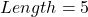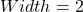Step-by-step explanation:

Given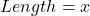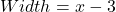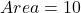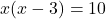See comment for missing part of the question

Required

Complete the expression to determine the dimension of a rectangle

We have:Open bracket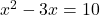Equate to 0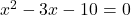Expand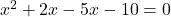Factorize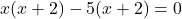Factor out x + 2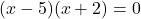Solve for x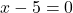or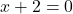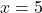or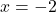The value of x cannot be negative

So: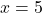Recall that: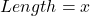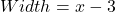So:—- i.e. 5 – 3